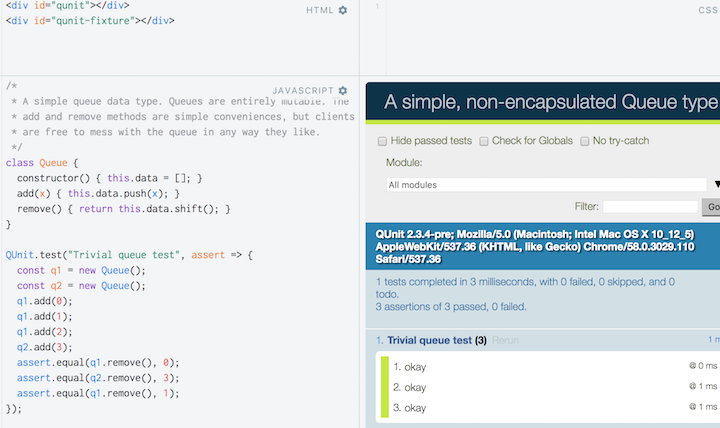# Unit Testing in JavaScript

Let’s test some JavaScript. We’ll see two frameworks: QUnit and Mocha.

## QUnit

QUnit is a JavaScript Unit Testing framework. It works well when running JavaScript in a browser, but isn’t limited to browser-based apps.

### Running QUint in JSFiddle

In the External Resources section, add the following URIs:

• `https://code.jquery.com/qunit/qunit-git.css`
• `https://code.jquery.com/qunit/qunit-git.js`

In the HTML section, enter:

```<div id="qunit"></div>
<div id="qunit-fixture"></div>
```

Write your tests and assertions according to the QUnit docs. Here’s a trivial example.

```/*
* A simple queue data type. Queues are entirely mutable.  The
* add and remove methods are simple conveniences, but clients
* are free to mess with the queue in any way they like.
*/
class Queue {
constructor() { this.data = []; }
remove() { return this.data.shift(); }
}

QUnit.test("Trivial queue test", assert => {
const q1 = new Queue();
const q2 = new Queue();
assert.equal(q1.remove(), 0);
assert.equal(q2.remove(), 3);
assert.equal(q1.remove(), 1);
});
```

Run the fiddle:### QUnit Assertions

The most common assertion methods you will use are:

• `assert.ok(condition, message)` passes if truthy
• `assert.NotOk(condition, message)` passes if falsy
• `assert.strictEqual(actual, expected, message)` passes if actual === expected
• `assert.notStrictEqual(actual, expected, message)` passes if actual !== expected
• `assert.deepEqual(actual, expected, message)` recurses `===` into object
• `assert.notDeepEqual(actual, expected, message)` fails if `deepEqual` passes
• `assert.throws(func, expected, message)` succeeds if func throws

Each of these, and all of the others, are explained in detail in the QUnit API Documentation.

### Running QUnit in CodePen

Here’s the same script as above, on CodePen. To get QUnit working, you have to add the QUnit JavaScript and CSS in the pen’s Settings.

See the Pen A trivial queue class with unit tests by Ray Toal (@rtoal) on CodePen.

### Running QUint outside the browser

There’s a neat little example of using QUint for standalone browser apps this way on the QUint home page.

There are also ways to run QUnit under Node.js, but we’ll learn how to do this with Mocha instead.

## Mocha

Mocha is the “simple, flexible, and fun” testing framework for JavaScript. It can run under Node.js and the browser.

Here’s the class we’ll test. Put in the file queue.js:

queue.js
```module.exports = class Queue {
constructor() { this.data = []; }
remove() { return this.data.shift(); }
size() { return this.data.length; }
};
```

Put the test in the file test/queue_tests.js:

queue_tests.js
```const Queue = require('../queue');
const assert = require('assert');

describe('A queue', () => {
it('should have a length of 0 upon creation', () => {
assert.equal(new Queue().size(), 0);
});

it('should should remove what was added', () => {
const q = new Queue();
assert.equal(q.remove(), 0);
assert.equal(q.remove(), 1);
});
});

describe('Each queue', () => {
it('should be distinct from any other', () => {
const q1 = new Queue();
const q2 = new Queue();
assert.equal(q2.size(), 0);
});
});
```

Run the test in the same folder as the class:

```\$ mocha

A queue
✓ should have a length of 0 upon creation
✓ should should remove what was added

Each queue
✓ should be distinct from any other

3 passing (6ms)
```

One fun thing about Mocha is the variety of reporters. Here are some in action:

```\$ mocha -R landing

----------------------------------------------------------------------------------------------
⋅⋅⋅⋅⋅⋅⋅⋅⋅⋅⋅⋅⋅⋅⋅⋅⋅⋅⋅⋅⋅⋅⋅⋅⋅⋅⋅⋅⋅⋅⋅⋅⋅⋅⋅⋅⋅⋅⋅⋅⋅⋅⋅⋅⋅⋅⋅⋅⋅⋅⋅⋅⋅⋅⋅⋅⋅⋅⋅⋅⋅⋅⋅⋅⋅⋅⋅⋅⋅⋅⋅⋅⋅⋅⋅⋅⋅⋅⋅⋅⋅⋅⋅⋅⋅⋅⋅⋅⋅⋅⋅⋅⋅⋅✈
----------------------------------------------------------------------------------------------

3 passing (5ms)
```
Exercise: Try `mocha -R list` and `mocha -R nyan`

Mocha runs just fine with the built-in `assert` module of Node.js (which we used above), or with any other assertion library such as Chai or Should.